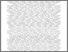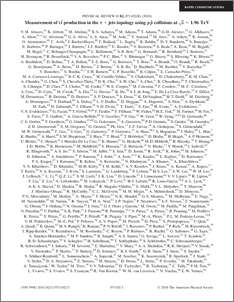# Measurement of ttbar production in the tau + jets topology using ppbar collisions at sqrt{s} = 1.96 TeV

Collaboration, D0 and Bertram, Iain and Borissov, Guennadi and Fox, Harald and Williams, Mark and Ratoff, Peter and Love, Peter and Sopczak, Andre (2010) Measurement of ttbar production in the tau + jets topology using ppbar collisions at sqrt{s} = 1.96 TeV. Physical Review D, 82 (7). ISSN 1550-7998Preview
PDF
PhysRevD.82.071102.pdf - Published Version

## Abstract

We present a measurement of the ttbar production cross section multiplied by the branching ratio to tau lepton decaying semihadronically plus jets, at a center of mass energy sqrt{s}=1.96 TeV using 1 fb^(-1) of integrated luminosity collected with the D0 detector. Assuming a top quark mass of 170 GeV, we measure sigma_ttb * BR=0.60^{+0.23}_{-0.22} (stat) ^{+0.15}_{-0.14} (syst) +/- 0.04\;(lumi) pb. In addition, we extract the ttbar production cross section using the ttbar->tau+jets topology, with the result sigma_ttbar = 6.9_{-1.2}^{+1.2} (stat)_{-0.7}^{+0.8} (syst) \pm 0.4 (lumi) pb. These findings are in good agreement with standard model predictions and measurements performed using other top quark decay channels.

Item Type:
Journal Article
Journal or Publication Title:
Physical Review D